# DAV Class 5 Maths Chapter 3 Worksheet 10 Solutions

The DAV Class 5 Maths Book Solutions Pdf and DAV Class 5 Maths Chapter 3 Worksheet 10 Solutions of Multiples and Factors offer comprehensive answers to textbook questions.

## DAV Class 5 Maths Ch 3 Worksheet 10 Solutions

Question 1.
Fill in the blanks.
(a) Prime factorisation of 15 : _______
Prime factorisation of 90 : _______
LCM of 15 and 90 = _______
Solution:
Prime factorisation of 15 : 3 × 5
Prime factorisation of 90 : 2 × 3 × 3 × 5
LCM of 15 and 90 = 2 × 3 × 3 × 5 = 90(b) Prime factorisation of 18 : _______
Prime factorisation of 24 : _______
LCM of 18 and 24 = _______
Solution:
Prime factorisation of 18 : 2 × 3 × 3
Prime factorisation of 24 : 2 × 2 × 2 × 3
LCM of 18 and 24 = 2 × 2 × 2 × 3 × 3 = 72(c) Prime factorisation of 25 : _______
Prime factorisation of 15 : _______
LCM of 25 and 15 = _______
Solution:
Prime factorisation of 25 : 5 × 5
Prime factorisation of 15 : 3 × 5
LCM of 25 and 15 = 5 × 5 × 3 = 75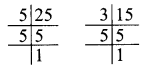(d) Prime factorisation of 27 : _______
Prime factorisation of 42 : _______
LCM of 27 and 42 = _______
Solution:
Prime factorisation of 27 : 3 × 3 × 3
Prime factorisation of 42 : 2 × 3 × 7
LCM of 27 and 42 = 3 × 3 × 3 × 2 × 7 = 378Question 2.
Using the prime factorisation method, find the LCM.
(a) 16 and 20
Solution:
16 and 20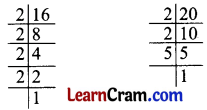Prime factorisation of 16 = 2 × 2 × 2 × 2
Prime factorisation of 20 = 2 × 2 × 5
LCM of 16 and 20 = 2 × 2 × 2 × 2 × 5 = 80

(b) 18 and 27
Solution:
18 and 27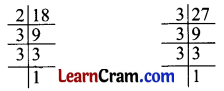Prime factorisation of 18 = 2 × 3 × 3
Prime factorisation of 27 = 3 × 3 × 3
LCM of 18 and 27 = 2 × 3 × 3 × 3 = 54

(c) 12 and 22
Solution:
12 and 22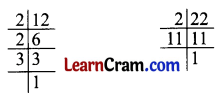Prime factorisation of 12 = 2 × 2 × 3
Prime factorisation of 22 = 2 × 11
LCM of 12 and 22 = 2 × 3 × 2 × 11 = 132(d) 15 and 24
Solution:
15 and 24Prime factorisation of 15 = 3 × 5
Prime factorisation of 24 = 2 × 2 × 2 × 3
LCM of 15 and 24 = 3 × 5 × 2 × 2 × 2 = 120

(e) 8 and 16
Solution:
8 and 16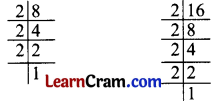Prime factorisation of 8 = 2 × 2 × 2
Prime factorisation of 16 = 2 × 2 × 2 × 2
LCM of 8 and 16 = 2 × 2 × 2 × 2 = 16

(f) 25 and 35
Solution:
25 and 35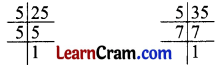Prime factorisation of 25 = 5 × 5
Prime factorisation of 35 = 5 × 7
LCM of 25 and 35 = 5 × 5 × 7 = 175(g) 36 and 45
Solution:
36 and 45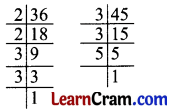Prime factorisation of 36 = 2 × 2 × 3 × 3
Prime factorisation of 45 = 3 × 3 × 5
LCM of 36 and 45 = 2 × 2 × 3 × 3 × 5 = 180

(h) 33 and 44
Solution:
33 and 44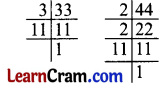Prime factorisation of 33 = 3 × 11
Prime factorisation of 44 = 2 × 2 × 11
LCM of 33 and 44 = 3 × 2 × 2 × 11 = 132

(i) 20 and 35
Solution:
20 and 35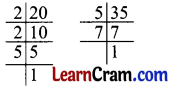Prime factorisation of 20 = 2 × 2 × 5
Prime factorisation of 35 = 5 × 7
LCM of 20 and 35 = 2 × 2 × 5 × 7 = 140(j) 54 and 38
Solution:
54 and 38Prime factorisation of 54 = 2 × 3 × 3 × 3
Prime factorisation of 38 = 2 × 19
LCM of 54 and 38 = 2 × 3 × 3 × 3 × 19 = 1026

DAV Class 5 Maths Chapter 3 Worksheet 10 Notes

Find the prime factorisation of 15, 35Thus, the LCM of 15 and 35 is 105.